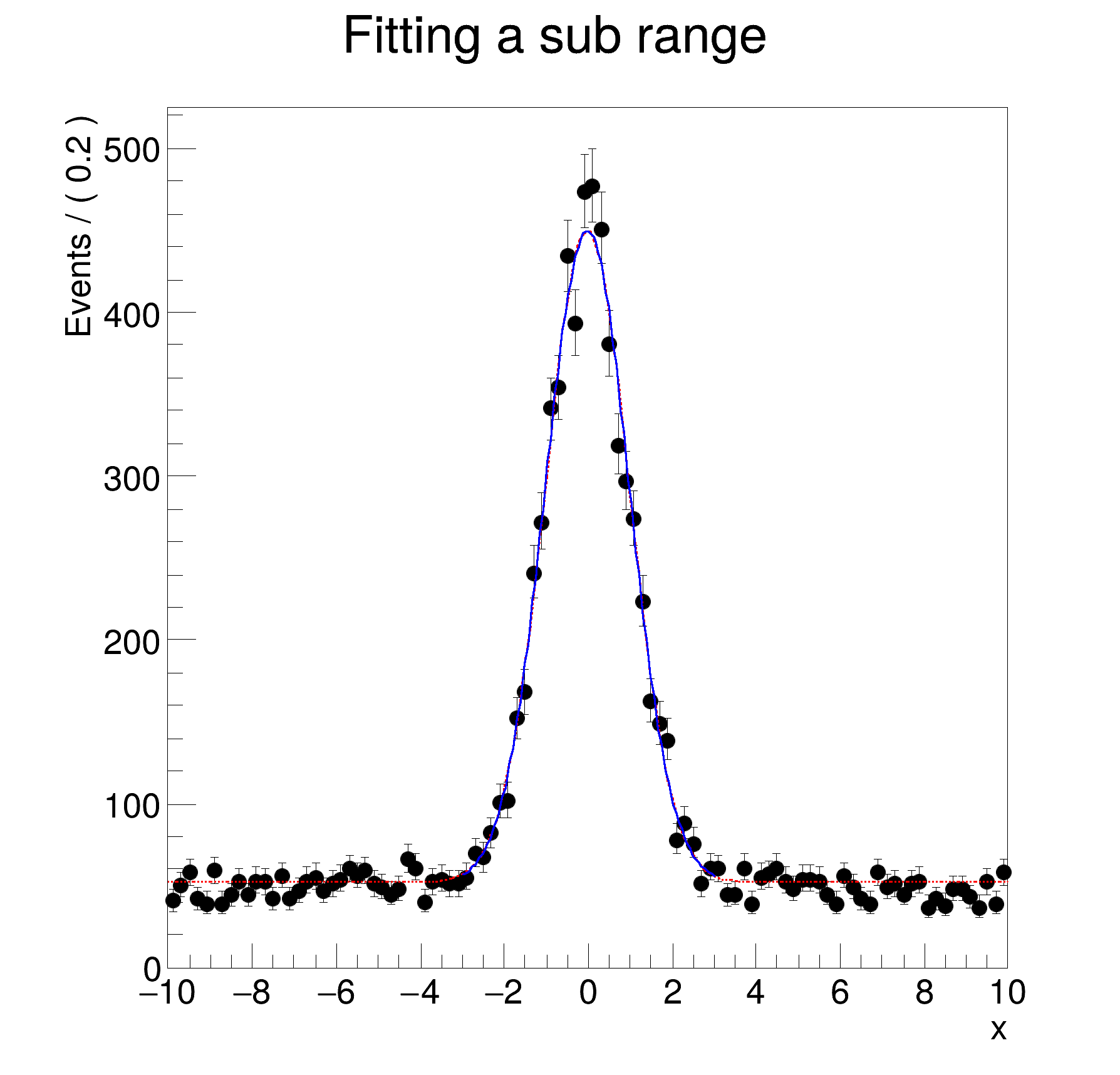ROOT   Reference Guide
Searching...
No Matches
rf203_ranges.C File Reference

## Detailed Description#include "RooRealVar.h"
#include "RooDataSet.h"
#include "RooGaussian.h"
#include "RooPolynomial.h"
#include "RooFitResult.h"
#include "RooPlot.h"
#include "TCanvas.h"
#include "TAxis.h"
#include "TH1.h"
using namespace RooFit;
{
// S e t u p m o d e l
// ---------------------
// Construct observables x
RooRealVar x("x", "x", -10, 10);
// Construct gaussx(x,mx,1)
RooRealVar mx("mx", "mx", 0, -10, 10);
RooGaussian gx("gx", "gx", x, mx, 1.0);
// Construct px = 1 (flat in x)
RooPolynomial px("px", "px", x);
// Construct model = f*gx + (1-f)px
RooRealVar f("f", "f", 0., 1.);
RooAddPdf model("model", "model", RooArgList(gx, px), f);
// Generated 10000 events in (x,y) from pdf model
std::unique_ptr<RooDataSet> modelData{model.generate(x, 10000)};
// F i t f u l l r a n g e
// ---------------------------
// Fit pdf to all data
std::unique_ptr<RooFitResult> r_full{model.fitTo(*modelData, Save(true), PrintLevel(-1))};
// F i t p a r t i a l r a n g e
// ----------------------------------
// Define "signal" range in x as [-3,3]
x.setRange("signal", -3, 3);
// Fit pdf only to data in "signal" range
std::unique_ptr<RooFitResult> r_sig{model.fitTo(*modelData, Save(true), Range("signal"), PrintLevel(-1))};
// P l o t / p r i n t r e s u l t s
// ---------------------------------------
// Make plot frame in x and add data and fitted model
RooPlot *frame = x.frame(Title("Fitting a sub range"));
modelData->plotOn(frame);
model.plotOn(frame, Range(""), LineStyle(kDashed), LineColor(kRed)); // Add shape in full ranged dashed
model.plotOn(frame); // By default only fitted range is shown
// Print fit results
cout << "result of fit on all data " << endl;
r_full->Print();
cout << "result of fit in in signal region (note increased error on signal fraction)" << endl;
r_sig->Print();
// Draw frame on canvas
new TCanvas("rf203_ranges", "rf203_ranges", 600, 600);
frame->GetYaxis()->SetTitleOffset(1.4);
frame->Draw();
return;
}
#define f(i)
Definition RSha256.hxx:104
@ kRed
Definition Rtypes.h:66
@ kDashed
Definition TAttLine.h:48
Option_t Option_t TPoint TPoint const char GetTextMagnitude GetFillStyle GetLineColor GetLineWidth GetMarkerStyle GetTextAlign GetTextColor GetTextSize void char Point_t Rectangle_t WindowAttributes_t Float_t Float_t Float_t Int_t Int_t UInt_t UInt_t Rectangle_t Int_t Int_t Window_t TString Int_t GCValues_t GetPrimarySelectionOwner GetDisplay GetScreen GetColormap GetNativeEvent const char const char dpyName wid window const char font_name cursor keysym reg const char only_if_exist regb h Point_t winding char text const char depth char const char mx
RooAddPdf is an efficient implementation of a sum of PDFs of the form.
RooArgList is a container object that can hold multiple RooAbsArg objects.
Definition RooArgList.h:22
Plain Gaussian p.d.f.
Definition RooGaussian.h:24
A RooPlot is a plot frame and a container for graphics objects within that frame.
Definition RooPlot.h:43
static RooPlot * frame(const RooAbsRealLValue &var, double xmin, double xmax, Int_t nBins)
Create a new frame for a given variable in x.
Definition RooPlot.cxx:239
TAxis * GetYaxis() const
Definition RooPlot.cxx:1279
void Draw(Option_t *options=nullptr) override
Draw this plot and all of the elements it contains.
Definition RooPlot.cxx:652
RooPolynomial implements a polynomial p.d.f of the form.
RooRealVar represents a variable that can be changed from the outside.
Definition RooRealVar.h:37
virtual void SetTitleOffset(Float_t offset=1)
Set distance between the axis and the axis title.
Definition TAttAxis.cxx:298
The Canvas class.
Definition TCanvas.h:23
RooCmdArg Save(bool flag=true)
RooCmdArg PrintLevel(Int_t code)
RooCmdArg LineColor(Color_t color)
RooCmdArg LineStyle(Style_t style)
Double_t x[n]
Definition legend1.C:17
The namespace RooFit contains mostly switches that change the behaviour of functions of PDFs (or othe...
const char * Title
Definition TXMLSetup.cxx:68
Ta Range(0, 0, 1, 1)
[#1] INFO:Minimization -- RooAbsMinimizerFcn::setOptimizeConst: activating const optimization
[#1] INFO:Minimization -- The following expressions have been identified as constant and will be precalculated and cached: (px)
[#1] INFO:Minimization -- The following expressions will be evaluated in cache-and-track mode: (gx)
[#1] INFO:Minimization -- RooAbsMinimizerFcn::setOptimizeConst: deactivating const optimization
[#1] INFO:Eval -- RooRealVar::setRange(x) new range named 'signal' created with bounds [-3,3]
[#1] INFO:Eval -- RooRealVar::setRange(x) new range named 'fit_nll_model_modelData' created with bounds [-3,3]
[#1] INFO:Fitting -- RooAbsOptTestStatistic::ctor(nll_model_modelData) constructing test statistic for sub-range named signal
[#1] INFO:Minimization -- RooAbsMinimizerFcn::setOptimizeConst: activating const optimization
[#1] INFO:Minimization -- The following expressions have been identified as constant and will be precalculated and cached: (px)
[#1] INFO:Minimization -- The following expressions will be evaluated in cache-and-track mode: (gx)
[#1] INFO:Minimization -- RooAbsMinimizerFcn::setOptimizeConst: deactivating const optimization
[#1] INFO:Plotting -- RooAbsPdf::plotOn(model) p.d.f was fitted in a subrange and no explicit NormRange() was specified. Plotting / normalising in fit range. To override, do one of the following
- Clear the automatic fit range attribute: <pdf>.removeStringAttribute("fitrange");
- Explicitly specify the plotting range: Range("<rangeName>").
- Explicitly specify where to compute the normalisation: NormRange("<rangeName>").
The default (full) range can be denoted with Range("") / NormRange("").
[#1] INFO:Plotting -- RooAbsPdf::plotOn(model) only plotting range ''
[#1] INFO:Plotting -- RooAbsPdf::plotOn(model) p.d.f. curve is normalized using explicit choice of ranges 'fit_nll_model_modelData'
[#1] INFO:Plotting -- RooAbsPdf::plotOn(model) p.d.f was fitted in a subrange and no explicit Range() and NormRange() was specified. Plotting / normalising in fit range. To override, do one of the following
- Clear the automatic fit range attribute: <pdf>.removeStringAttribute("fitrange");
- Explicitly specify the plotting range: Range("<rangeName>").
- Explicitly specify where to compute the normalisation: NormRange("<rangeName>").
The default (full) range can be denoted with Range("") / NormRange("").
[#1] INFO:Plotting -- RooAbsPdf::plotOn(model) only plotting range 'fit_nll_model_modelData'
[#1] INFO:Plotting -- RooAbsPdf::plotOn(model) p.d.f. curve is normalized using explicit choice of ranges 'fit_nll_model_modelData'
result of fit on all data
RooFitResult: minimized FCN value: 25939.4, estimated distance to minimum: 3.77183e-06
covariance matrix quality: Full, accurate covariance matrix
Status : MINIMIZE=0 HESSE=0
Floating Parameter FinalValue +/- Error
-------------------- --------------------------
f 5.0441e-01 +/- 6.32e-03
mx -2.1605e-02 +/- 1.77e-02
result of fit in in signal region (note increased error on signal fraction)
RooFitResult: minimized FCN value: 10339.5, estimated distance to minimum: 0.000279216
covariance matrix quality: Full, accurate covariance matrix
Status : MINIMIZE=0 HESSE=0
Floating Parameter FinalValue +/- Error
-------------------- --------------------------
f 4.8979e-01 +/- 1.62e-02
mx -2.1518e-02 +/- 1.79e-02
Date
July 2008

Definition in file rf203_ranges.C.